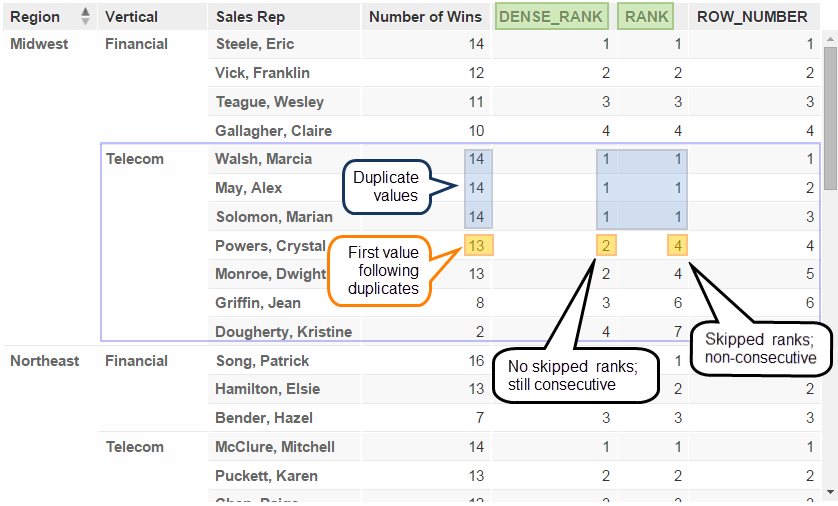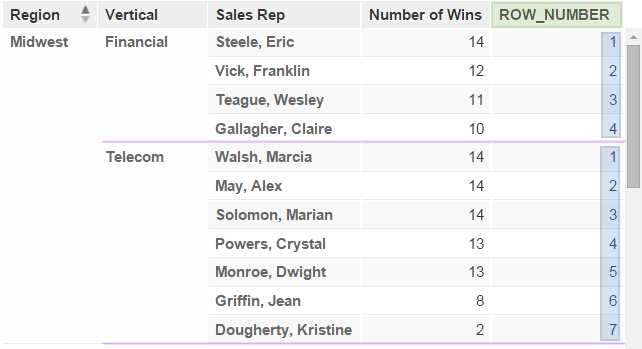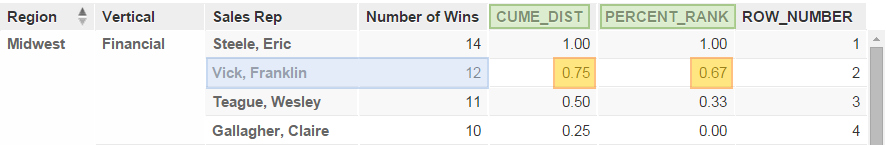# Rank Variations

MAQL includes the following ranking functions that sequentially rank all values in a report.

The following ranking functions differ from the TOP(n) and BOTTOM(n) filters which filter only for a specified number of top or bottom values (such as the top five or bottom five sales reps).

Function

Definition

RANK

Assigns a rank to each value in a set of values, leaving gaps between rows of duplicate values (ties) within the set. If any values within a set are the same (tied), the next rank following the tie is skipped, resulting in non-consecutive ranking.

DENSE_RANK

Assigns a rank to each value in a set of values, leaving no gaps between rows of duplicate values (ties) within the set. If any values within a set are the same (tied), the next rank following the tie is not skipped and the ranking remains consecutive.

ROW_NUMBER

Assigns a unique row number, starting from 1, to every row within a set of values, regardless of duplicate values (ties) within the set.

PERCENT_RANK

In a set of values, calculates the number of values that are less than the current value, minus 1, divided by the total number of values in the set, minus 1. The first value in any set has a PERCENT_RANK of zero.  (relative rank of the current value - 1) / (number of values in the set - 1)

CUME_DIST

In a set of values, calculates the number of values that are less than or equal to the current value (inclusive), divided by the total number of values in the set (cumulative distribution). ( relative rank of the current value) / (number of values in the set)

## RANK and DENSE_RANK

Though similar, the RANK and DENSE_RANK functions differ in the way each ranks the first row that follows rows of duplicate values.

In the following illustration, see how the output of RANK skips ranks (leaves a gap), whereas DENSE_RANK does not skip ranks (leaves no gap).• RANK identifies Crystal Powers as the fourth-highest ranking Sales Rep in terms of number of wins in Telecom, Midwest.

``````SELECT RANK (#WINS) DESC WITHIN(Region, Vertical)
``````
• DENSE_RANK identifies Crystal Powers as the Sales Rep with the second-highest number of wins in Telecom, Midwest.

``````SELECT DENSE_RANK (#WINS) DESC WITHIN(Region, Vertical)
``````

## ROW_NUMBER

The ROW_NUMBER function assigns a unique number to every row in a set, regardless of duplicate values within the set.

``````SELECT ROW_NUMBER(#WINS) DESC WITHIN(Region, Vertical)
``````

The following illustration shows how ROW_NUMBER can be used with the WITHIN clause (see WITHIN Clause) to return unique row numbers for several subgroups in a report. The presence of duplicate values (ties) in the Number of Wins column has no effect on ROW_NUMBER.## CUME_DIST and PERCENT_RANK

The CUME_DIST and PERCENT_RANK functions are very similar.

The following statements clarify how they differ.

• CUME_DIST (cumulative distribution) returns a number greater than 0 and up to and including 1 which represents the relative position of the value in the specified row within a group of N rows. In the following figure, the CUME_DIST calculation shows that 75% of sales reps in Financial, Midwest have Number of Wins that are less than or equal to those of Franklin Vic.

``````SELECT CUME_DIST (#WINS) WITHIN(Region, Vertical)
``````
• PERCENT_RANK returns a number from 0 to 1 (inclusive) which represents the relative position of the specified row, minus 1, within a group of N rows, minus 1. In the following figure, the PERCENT_RANK calculation shows that Franklin Vic is at the 67% percentile in terms of total Number of Wins in his group.

``````SELECT PERCENT_RANK (#WINS) WITHIN(Region, Vertical)
``````